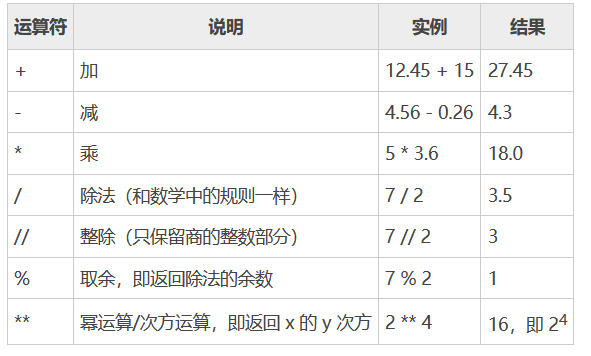### 文章目录

• 前言
• 一，+ 加法运算符
• 请看代码：
• 运行结果：
• 拼接字符串
• 请看代码：
• 运行结果：
• 二，- 减法运算符
• 请看代码：
• 运行结果：
• 求负
• 请看代码：
• 运行结果：
• 注意，单独使用+是无效的，不会改变数字的值。
• 例如：
• 运行结果：
• 三，＊乘法运算符
• 请看代码：
• 运行结果：
• 重复字符串
• 请看代码：
• 运行结果：
• 四，/ 和 // 除法运算符
• 请看代码：
• 运行结果：
• Python 2.x 中的除法
• 请看代码：
• 运行结果：
• 五，% 求余运算符
• Python % 使用示例：
• 运行结果：
• 六，** 次方（乘方）运算符
• Python ** 运算符示例：
• 运行结果：

# 前言## 一，+ 加法运算符

#### 请看代码：

``````    m = 10
n = 97
sum1 = m + n
x = 7.2
y = 15.3
sum2 = x + y
print("sum1=%d, sum2=%.2f" % (sum1, sum2) )
``````
• 1
• 2
• 3
• 4
• 5
• 6
• 7

#### 运行结果：

``````sum1=107, sum2=22.50
``````
• 1

### 拼接字符串

#### 请看代码：

``````name = "C语言中文网"
url = "http://c.biancheng.net/"
age = 8
info = name + "的网址是" + url + "，它已经" + str(age) + "岁了。"
print(info)
``````
• 1
• 2
• 3
• 4
• 5

#### 运行结果：

``````C语言中文网的网址是http://c.biancheng.net/，它已经8岁了。
str() 函数用来将整数类型的 age 转换成字符串。
``````
• 1
• 2

## 二，- 减法运算符

#### 请看代码：

``````n = 45
m = -n
x = -83.5
y = -x
print(m, ",", y)
``````
• 1
• 2
• 3
• 4
• 5

#### 运行结果：

``````-45 , 83.5
``````
• 1

### 求负

#### 请看代码：

``````n = 45
n_neg = -n
f = -83.5
f_neg = -f
print(n_neg, ",", f_neg)
``````
• 1
• 2
• 3
• 4
• 5

#### 运行结果：

``````-45 , 83.5
``````
• 1

### 注意，单独使用+是无效的，不会改变数字的值。

#### 例如：

``````n = 45
m = +n
x = -83.5
y = +x
print(m, ",", y)
``````
• 1
• 2
• 3
• 4
• 5

#### 运行结果：

``````45 , -83.5
``````
• 1

## 三，＊乘法运算符

#### 请看代码：

``````n = 4 * 25
f = 34.5 * 2
print(n, ",", f)
``````
• 1
• 2
• 3

#### 运行结果：

``````100 , 69.0
``````
• 1

### 重复字符串

#### 请看代码：

``````str1 = "hello "
print(str1 * 4)
``````
• 1
• 2

#### 运行结果：

``````hello hello hello hello
``````
• 1

## 四，/ 和 // 除法运算符

Python 支持/和//两个除法运算符，但它们之间是有区别的：

• /表示普通除法，使用它计算出来的结果和数学中的计算结果相同。
• //表示整除，只保留结果的整数部分，舍弃小数部分；注意是直接丢掉小数部分，而不是四舍五入。

#### 请看代码：

``````#整数不能除尽
print("23/5 =", 23/5)
print("23//5 =", 23//5)
print("23.0//5 =", 23.0//5)
print("-------------------")
#整数能除尽
print("25/5 =", 25/5)
print("25//5 =", 25//5)
print("25.0//5 =", 25.0//5)
print("-------------------")
#小数除法
print("12.4/3.5 =", 12.4/3.5)
print("12.4//3.5 =", 12.4//3.5)
``````
• 1
• 2
• 3
• 4
• 5
• 6
• 7
• 8
• 9
• 10
• 11
• 12
• 13

#### 运行结果：

``````23/5 = 4.6
23//5 = 4
23.0//5 = 4.0
-------------------
25/5 = 5.0
25//5 = 5
25.0//5 = 5.0
-------------------
12.4/3.5 = 3.542857142857143
12.4//3.5 = 3.0
``````
• 1
• 2
• 3
• 4
• 5
• 6
• 7
• 8
• 9
• 10

• /的计算结果总是小数，不管是否能除尽，也不管参与运算的是整数还是小数。
• 当有小数参与运算时，//结果才是小数，否则就是整数。

### Python 2.x 中的除法

Python 2.x 只提供了一种除法运算，就是/，它的行为和大部分编程语言中/的行为是一样的：

• 当/两边都是整数时，结果始终是整数；如果不能除尽，就直接舍弃小数部分。
• 当/两边有一个是小数时，结果始终是小数；如果恰好除尽，小数部分就是 0。

#### 请看代码：

``````#整数除法
print "18/6 =", 18/6
print "47/7 =", 47/7
print "----------------"
#小数除法
print "18.0/6 =", 18.0/6
print "47.0/7 =", 47.0/7
print "29.5/4.2 =", 29.5/4.2
``````
• 1
• 2
• 3
• 4
• 5
• 6
• 7
• 8

#### 运行结果：

``````18/6 = 3
47/7 = 6
----------------
18.0/6 = 3.0
47.0/7 = 6.71428571429
29.5/4.2 = 7.02380952381
``````
• 1
• 2
• 3
• 4
• 5
• 6

## 五，% 求余运算符

Python % 运算符用来求得两个数相除的余数，包括整数和小数。Python 使用第一个数字除以第二个数字，得到一个整数的商，剩下的值就是余数。对于小数，求余的结果一般也是小数。

### Python % 使用示例：

``````print("-----整数求余-----")
print("15%6 =", 15%6)
print("-15%6 =", -15%6)
print("15%-6 =", 15%-6)
print("-15%-6 =", -15%-6)
print("-----小数求余-----")
print("7.7%2.2 =", 7.7%2.2)
print("-7.7%2.2 =", -7.7%2.2)
print("7.7%-2.2 =", 7.7%-2.2)
print("-7.7%-2.2 =", -7.7%-2.2)
print("---整数和小数运算---")
print("23.5%6 =", 23.5%6)
print("23%6.5 =", 23%6.5)
print("23.5%-6 =", 23.5%-6)
print("-23%6.5 =", -23%6.5)
print("-23%-6.5 =", -23%-6.5)
``````
• 1
• 2
• 3
• 4
• 5
• 6
• 7
• 8
• 9
• 10
• 11
• 12
• 13
• 14
• 15
• 16

### 运行结果：

``````-----整数求余-----
15%6 = 3
-15%6 = 3
15%-6 = -3
-15%-6 = -3
-----小数求余-----
7.7%2.2 = 1.0999999999999996
-7.7%2.2 = 1.1000000000000005
7.7%-2.2 = -1.1000000000000005
-7.7%-2.2 = -1.0999999999999996
---整数和小数运算---
23.5%6 = 5.5
23%6.5 = 3.5
23.5%-6 = -0.5
-23%6.5 = 3.0
-23%-6.5 = -3.5
``````
• 1
• 2
• 3
• 4
• 5
• 6
• 7
• 8
• 9
• 10
• 11
• 12
• 13
• 14
• 15
• 16

• 只有当第二个数字是负数时，求余的结果才是负数。换句话说，求余结果的正负和第一个数字没有关系，只由第二个数字决定。
• %两边的数字都是整数时，求余的结果也是整数；但是只要有一个数字是小数，求余的结果就是小数。

## 六，** 次方（乘方）运算符

Python ** 运算符用来求一个 x 的 y 次方，也即次方（乘方）运算符。

### Python ** 运算符示例：

``````print('----次方运算----')
print('3**4 =', 3**4)
print('2**5 =', 2**5)
print('----开方运算----')
print('81**(1/4) =', 81**(1/4))
print('32**(1/5) =', 32**(1/5))
``````
• 1
• 2
• 3
• 4
• 5
• 6

### 运行结果：

``````----次方运算----
3**4 = 81
2**5 = 32
----开方运算----
81**(1/4) = 3.0
32**(1/5) = 2.0
``````
• 1
• 2
• 3
• 4
• 5
• 6